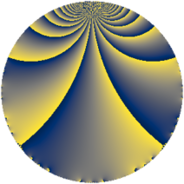# Properties

 Label 171.2.mLevel $171$ Weight $2$ Character orbit 171.m Rep. character $\chi_{171}(8,\cdot)$ Character field $\Q(\zeta_{6})$ Dimension $16$ Newform subspaces $1$ Sturm bound $40$ Trace bound $0$

# Related objects

## Defining parameters

 Level: $$N$$ $$=$$ $$171 = 3^{2} \cdot 19$$ Weight: $$k$$ $$=$$ $$2$$ Character orbit: $$[\chi]$$ $$=$$ 171.m (of order $$6$$ and degree $$2$$) Character conductor: $$\operatorname{cond}(\chi)$$ $$=$$ $$57$$ Character field: $$\Q(\zeta_{6})$$ Newform subspaces: $$1$$ Sturm bound: $$40$$ Trace bound: $$0$$

## Dimensions

The following table gives the dimensions of various subspaces of $$M_{2}(171, [\chi])$$.

Total New Old
Modular forms 48 16 32
Cusp forms 32 16 16
Eisenstein series 16 0 16

## Trace form

 $$16q - 16q^{4} + 8q^{7} + O(q^{10})$$ $$16q - 16q^{4} + 8q^{7} + 12q^{10} - 24q^{13} - 24q^{16} - 12q^{19} + 24q^{22} + 20q^{25} - 12q^{28} + 12q^{34} - 24q^{40} + 12q^{52} - 4q^{55} + 128q^{58} - 44q^{61} + 168q^{64} - 24q^{67} - 168q^{70} - 20q^{73} - 96q^{76} - 48q^{79} + 28q^{82} - 56q^{85} - 24q^{91} + 48q^{97} + O(q^{100})$$

## Decomposition of $$S_{2}^{\mathrm{new}}(171, [\chi])$$ into newform subspaces

Label Dim. $$A$$ Field CM Traces $q$-expansion
$$a_2$$ $$a_3$$ $$a_5$$ $$a_7$$
171.2.m.a $$16$$ $$1.365$$ $$\mathbb{Q}[x]/(x^{16} + \cdots)$$ None $$0$$ $$0$$ $$0$$ $$8$$ $$q-\beta _{1}q^{2}+(-\beta _{3}+2\beta _{4}+\beta _{10})q^{4}+\cdots$$

## Decomposition of $$S_{2}^{\mathrm{old}}(171, [\chi])$$ into lower level spaces

$$S_{2}^{\mathrm{old}}(171, [\chi]) \cong$$ $$S_{2}^{\mathrm{new}}(57, [\chi])$$$$^{\oplus 2}$$